IE时代的Matrix滤镜

filter: progid:DXImageTransform.Microsoft.Matrix( SizingMethod= sMethod ,  M11= fM11 , M12= fM12 , M21= fM21 , M22= fM22 )

了解css3动画之方法

css3为我们提供了2大属性Transform和animation（这个属性我会在另外一篇文章专门探究）

移动translate

transform:translate(30px,30px)

缩放scale

transform:scale(0.5)

旋转rotate

transform:rotate(90deg)

扭曲skew

transform:skew(30deg，30deg)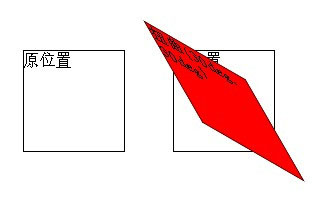矩阵变形matrix

#位移变换：
transform: translate(30px,30px);
transform: matrix(1, 0, 0, 1, 30, 30);
#缩放变换：
transform: scale(0.5);
transform: matrix(0.5, 0, 0, 0.5, 0, 0);
#旋转变换
transform: rotate(45deg);
transform: matrix(0.53, 0.85, -0.85, 0.53, 0, 0);//matrix(cos45',sin45',-sin45',cos45',0,0);
#扭曲变换
transform: skew(45deg);
transform: matrix(1,0,1,1,0,0);//tan45'=1;matrix(1,tan45',tan45',1,0,0)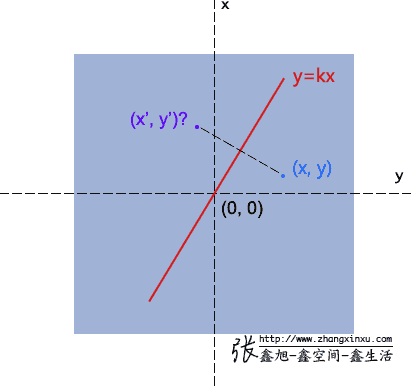(图来自 [张鑫旭的博客])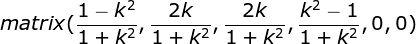深入css3动画之实现

知识准备

矩阵的乘积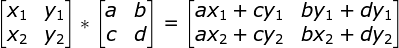几何向量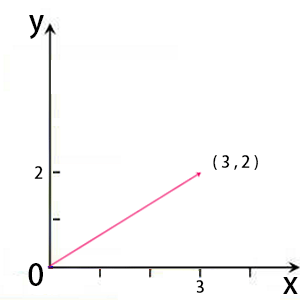通过矩阵求解缩放、扭曲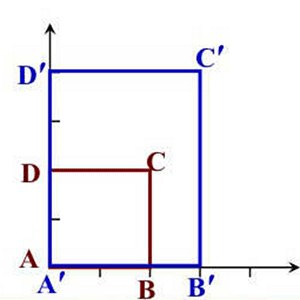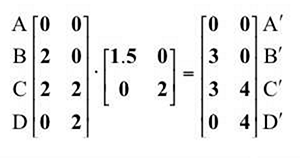y轴的变换向量为（0，1），你可以理解为缩放大小不变，同时没有扭曲，聪明你的是否立马能想到如何让高度也扭曲了吗？那么那你也一定知道如何让它同时缩放又扭曲

#扭曲变换
transform: skew(45deg);
transform: matrix(1,0,1,1,0,0);//matrix(a,b,c,d,0,0)，请按照矩阵图一一对应下

通过矩阵求解旋转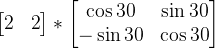x`=x+l;
y`=y+m;

总结一下全文高潮部分(复杂变换求解)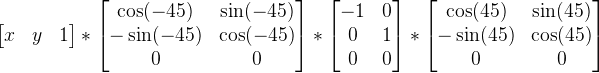观后思考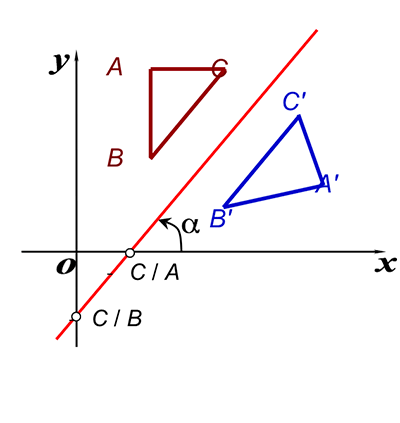「五年博客，如果觉得我的文章对您有用，请帮助本站成长」